# Measurement

When we measure, we use measurings tool to compare some dimension of an object to a standard unit. We use tools such as a thermometer, ruler, watch and scale etc.. to help measure different quantities.

## Measuring

We use either the Metric or US standard when making measurements.

The US standard, which is exclusively used within the United States, uses units such as: cups, pints, quarts and gallons for measuring liquid capacity, ounces, pounds and tons to measure weight, and inches, feet, yards and miles to measure distance.

The Metric system, which is widely shared among the rest of the world, uses units such as mililiter and liter for measuring liquid capacity, grams, kilogram and tonnes for measuring weight and centimeter, meter and kilometer to measure distance.

What are some of the possible units used to measure each of the following? Click to reveal the answers.

Length:   meter, feet, inch, km

Volume:   mL, cup, teaspoon

Weight:   pounds, grams

Temperature:   Celcius, Farenheit

## Units of Measure

Different units can be used to measure the same quantity (such as feet and meter for distance).

Try to match the following units of measurement to their correct description.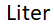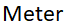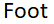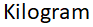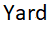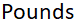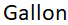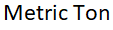## Unit Conversions

Since different units can be used, we may need to convert a value to another unit.

A conversion factor is a ratio (or a fraction) which represents the relationship between two different units. The quantity on the numerator is equal to the quantity on the denominator. Thus, a conversion factor is always be equal to 1 (even if the numbers are different!).

For example: $$\cfrac{7 \ \text{days}}{1 \ \text{week}}, \cfrac{100 \ \text{cm}}{1\ \text{m}}, \cfrac{1000 \ \text{mL}}{1 \ \text{L}}$$

Below are some common unit conversions.

Length Time Volume
1 kilometer = 1,000 meters 1 minute = 60 seconds 1 acre = 43,560 square feet
1 foot = 0.3048 meter 1 hour = 60 minutes 1 acre= 4,046 square meters
1 mile = 5,280 feet 1 day = 24 hours 1 square mile = 640 acres
1 foot = 12 inches 1 week = 7 days 1 square yard = 9 square foot

It takes about 3 days to get to the moon. If a movie is on average about 120 minutes, how many movies do you need to bring a long on a trip to the moon?

Since the units are different (days and minutes) we need to convert one quantity so that the units are the same. To do this, we will need a unit conversion. From above we know that 1 day is 24 hours and in 1 hour, there are 60 minutes. Thus:

$$1 \; \text{day} = 24 \; \text{hour} \cdot 60 \; \text{minute} = 1,440 \; \text{minutes}$$

To convert 3 days into minutes, first start with 3 days and the conversion factor 1 day = 1,440 minutes. We will set up the conversion as a multiplication but need the final unit (minutes) to be on top and the other unit (days) to cancel:

$$3 \; \text{days} \cdot \cfrac{1,440 \; \text{minutes}}{1 \; \text{days}} = 4,320 \; \text{minutes}$$

Notice that the days cancel and we are left with minutes - the desired unit.

If 3 days is 4,320 minutes and each movie is 120 minutes, the number of movies we need is:

$$\cfrac{4320}{120} = 36$$

Convert 12,000 m to km.

Convert 60 km/h (kilometers per hour) to m/s (meters per second)